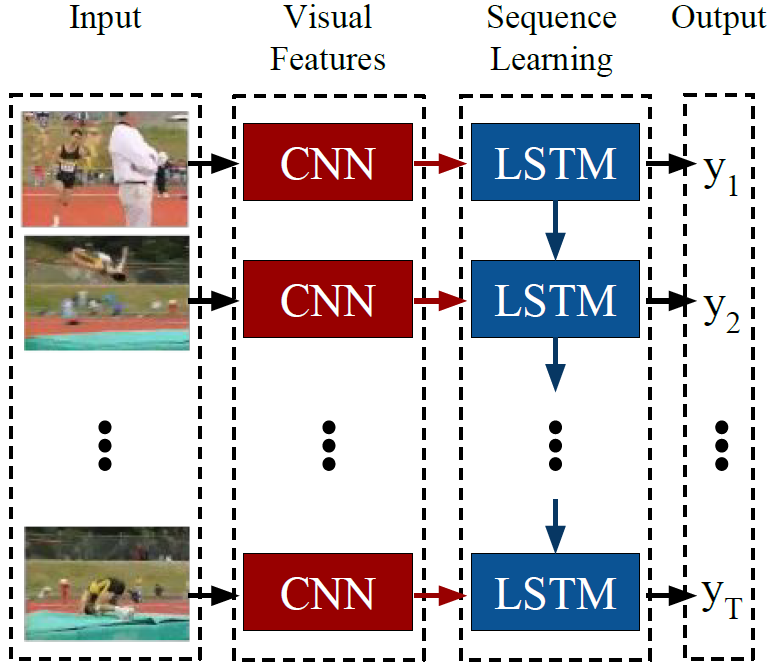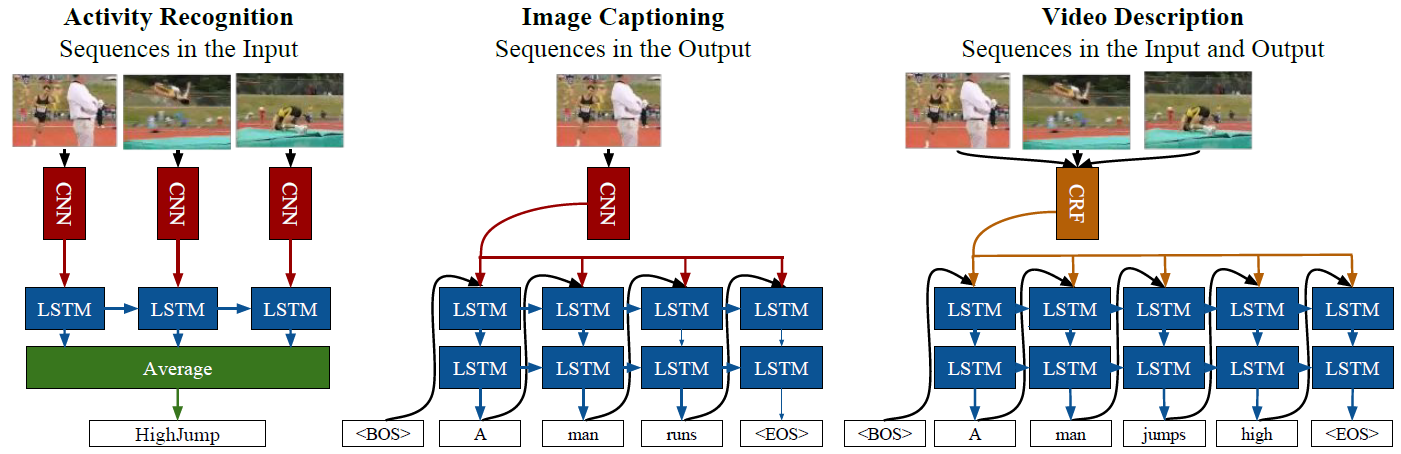There has been a lot of attempt to combine between Convolutional Neural Network (CNN) and Recurrent Neural Network (RNN) for image-based sequence recognition or video classification tasks. Today, we are going to see one of the combination between CNN and RNN for video classification tasks and how to implement it in Keras.

## Long-term Recurrent Convolutional Network (LRCN)

The Long-term Recurrent Convolutional Network (LRCN) is proposed by Jeff Donahue et al. in 2016. It is a combination of CNN and RNN, end-to-end trainable and suitable for large-scale visual understanding tasks such as video description, activity recognition and image captioning. The recurrent convolutional models are deeper in that they learn compositional representations in space and time, when the previous models assumed a fixed visual representation or perform simple temporal averaging for sequential processing. And it can be trained or learn temporal dynamics and convolutional perceptual representations as it is directly connected to convolutional network.Above figure is an example architecture of LRCN. As it is described in the figure, LRCN processes the variable-length visual input with a CNN. And their outputs are fed into a stack of recurrent sequence models which is LSTM in the figure. The final output from the sequence models is a variable-length prediction. This makes LRCN is proper models to handle tasks with time-varying inputs and output, such as activity recognition, image captioning and video description. Below figure is task-specific instantiations of LRCN model for each task.## Implementation in Keras

In the LCRN paper, the implementation of LRCN model is done by using Caffe which is a famous deep learning framework. But in this post, we are going to see how it can be written in Keras. We have to use TimeDistributed wrapper in order to apply a layer to every temporal slice an input. It allows us to distribute layers of a CNN across an time dimension. The CNN part of the model can be used any convolutional neural network model, but we have to think about trade-off between size of the model and memory space. The RNN part of the model is done by using LSTM model, which is one of the most used RNN model.

### Example code of LRCN in Keras


def LRCN(self):

model = Sequential()

kernel_initializer="he_normal", activation='relu')))